### ASM to Sega Genesis Platform

Would you like to react to this message? Create an account in a few clicks or log in to continue.
ASM to Sega Genesis Platform

All about assembly programming in the Sega Genesis console.

3 posters

# Homing Attack in Sonic 1 - Step 3 - Distance of Homing AttackOuricoDoido

Mensagens : 46
Data de inscrição : 2011-01-09
Current Project : Sonic Open Source Project

As you may have noticed, Sonic is attacking at any distance, and to corret this,
we need add a distance calculator using the Pythagorean theorem, because the Homing Attack is 360º.

go to HA_calcdistance and you see:
Code:
`HA_calcdistance:      move.w  8(a1),d1   ; move the object x-position to d1      move.w  \$C(a1),d2   ; move the object y-position to d2      sub.w  8(a0),d1   ; sub sonic x-position of object x-position      sub.w  \$C(a0),d2   ; sub sonic y-position of object y-position`
Code:
`      muls.w  d1,d1   ; horizontal distance squared      muls.w  d2,d2   ; vertical distance squared      add.l  d2,d1   ; add vertical distance to horizontal distance      cmp.l  #16384,d1      ; is distance of Sonic, greater than or equal 128 pixels of the object? (128^2=16384 // \$80^2=\$4000)      bge.s  HA_nextobject   ; if yes, don't execute the homing attack`
This is the Pythagorean theorem (a^2=b^2+c^2)
D1 and D2 are the leg b and c, and the "16384" is the predetermined "a^2".
To change the distance by another value, change the #16384 (or \$4000) by another number n^2.

But if you compile and test the ROM, you see that Homing Attack don't work correctly.
Why? Because the CalcAngle use the d1 & d2, and in these lines of code, the d1 & d2 are changed (multiplied), what cause the error.
How to correct this? Recalculating the value is a good option.

Code:
`; ---------------------------------------------------------------------------; Recalculating the distance between the Sonic and the object (d1 = x distance / d2 = y distance)      move.w  8(a1),d1   ; move the object x-position to d1      move.w  \$C(a1),d2   ; move the object y-position to d2      sub.w  8(a0),d1   ; sub sonic x-position of object x-position      sub.w  \$C(a0),d2   ; sub sonic y-position of object y-position`
Why d1 and d2?
Becuse the CalcAngle uses d1 and d2 as entry points.

Why not d0?
If you want to use the D0, you can use instead of d1, d2, but CalcAngle uses d1 and d2 as entry points.

Homing Attack only work if Sonic is facing the object

As you may have noticed, Sonic is attacking the object without be facing for him.
To correct this, we need test if the Sonic is facing the object

Below this:
Code:
`HA_calcdistance:   ; distance calculator      move.w  8(a1),d0   ; move the object x-position to d0      move.w  \$C(a1),d1   ; move the object y-position to d1      sub.w  8(a0),d0   ; sub sonic x-position of object x-position      sub.w  \$C(a0),d1   ; sub sonic y-position of object y-position`
Code:
`; ---------------------------------------------------------------------------; test if the Sonic is facing the object      btst    #0,\$22(a0)   ; is sonic facing left?      beq.s  HA_faceleft   ; if yes, branch      cmpi.w  #8,d1      ; is distance of Sonic, less than 8 pixels of the object?      blt.s  HA_calcdistance2   ; if yes, branch      bra.s  HA_nextobjectHA_faceleft:      cmpi.w  #-8,d1      ; is distance of Sonic, greater than -8 pixels of the object?      bgt.s  HA_calcdistance2   ; if yes, branch      bra.s  HA_nextobject; end of test; ---------------------------------------------------------------------------HA_calcdistance2:      ; continuation of distance calculator`
What is the "8" and the "-8"?
Is the maximum distance that the Sonic can attack the object, without be facing for him.

The basic Homig Attack is completeGreen Snake

Mensagens : 2185
Data de inscrição : 2012-04-07
Localização : I do not even know
Current Project : nopeJdpense

Mensagens : 100278
Data de inscrição : 2014-08-21
Just an annual year bump!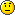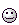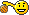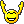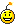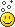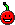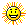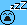Green Snake

Mensagens : 2185
Data de inscrição : 2012-04-07
Localização : I do not even know
Current Project : nope